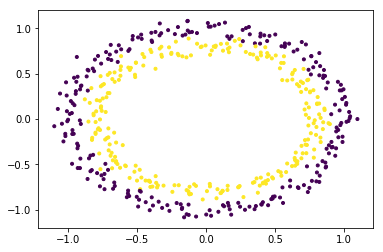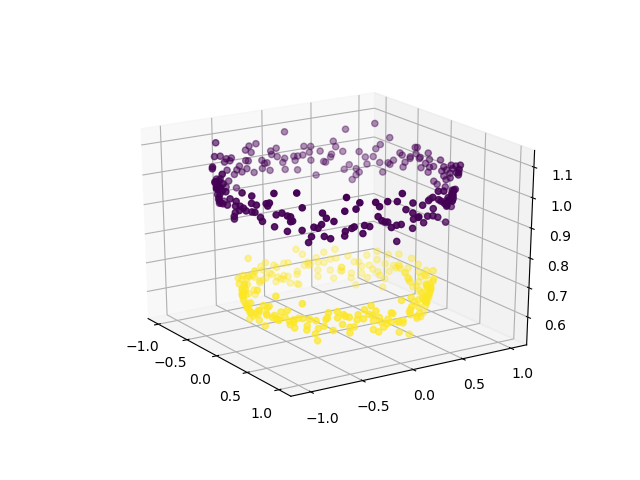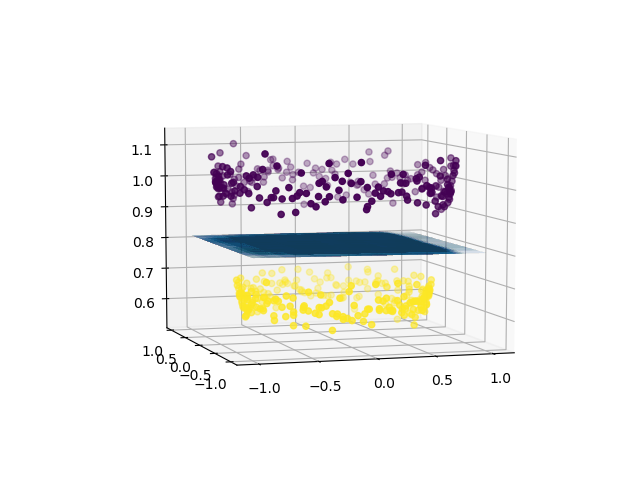Related Articles
ML | Using SVM to perform classification on a non-linear dataset
• Last Updated : 15 Jan, 2019

Prerequisite: Support Vector Machines

Definition of a hyperplane and SVM classifier:
For a linearly separable dataset having n features (thereby needing n dimensions for representation), a hyperplane is basically an (n – 1) dimensional subspace used for separating the dataset into two sets, each set containing data points belonging to a different class. For example, for a dataset having two features X and Y (therefore lying in a 2-dimensional space), the separating hyperplane is a line (a 1-dimensional subspace). Similarly, for a dataset having 3-dimensions, we have a 2-dimensional separating hyperplane, and so on.
In machine learning, Support Vector Machine (SVM) is a non-probabilistic, linear, binary classifier used for classifying data by learning a hyperplane separating the data.

Classifying a non-linearly separable dataset using a SVM – a linear classifier:
As mentioned above SVM is a linear classifier which learns an (n – 1)-dimensional classifier for classification of data into two classes. However, it can be used for classifying a non-linear dataset. This can be done by projecting the dataset into a higher dimension in which it is linearly separable!

To get a better understanding, let’s consider circles dataset.

 `# importing libraries``import` `numpy as np``import` `matplotlib.pyplot as plt``from` `sklearn.datasets ``import` `make_circles``from` `mpl_toolkits.mplot3d ``import` `Axes3D`` ` `# generating data``X, Y ``=` `make_circles(n_samples ``=` `500``, noise ``=` `0.02``)`` ` `# visualizing data``plt.scatter(X[:, ``0``], X[:, ``1``], c ``=` `Y, marker ``=` `'.'``)``plt.show()`The dataset is clearly a non-linear dataset and consists of two features (say, X and Y).

In order to use SVM for classifying this data, introduce another feature Z = X2 + Y2 into the dataset. Thus, projecting the 2-dimensional data into 3-dimensional space. The first dimension representing the feature X, second representing Y and third representing Z (which, mathematically, is equal to the radius of the circle of which the point (x, y) is a part of). Now, clearly, for the data shown above, the ‘yellow’ data points belong to a circle of smaller radius and the ‘purple’ data points belong to a circle of larger radius. Thus, the data becomes linearly separable along the Z-axis.

 `# adding a new dimension to X``X1 ``=` `X[:, ``0``].reshape((``-``1``, ``1``))``X2 ``=` `X[:, ``1``].reshape((``-``1``, ``1``))``X3 ``=` `(X1``*``*``2` `+` `X2``*``*``2``)``X ``=` `np.hstack((X, X3))`` ` `# visualizing data in higher dimension``fig ``=` `plt.figure()``axes ``=` `fig.add_subplot(``111``, projection ``=` `'3d'``)``axes.scatter(X1, X2, X1``*``*``2` `+` `X2``*``*``2``, c ``=` `Y, depthshade ``=` `True``)``plt.show()`Now, we can use SVM (or, for that matter, any other linear classifier) to learn a 2-dimensional separating hyperplane. This is how the hyperplane would look like:

 `# create support vector classifier using a linear kernel``from` `sklearn ``import` `svm`` ` `svc ``=` `svm.SVC(kernel ``=` `'linear'``)``svc.fit(X, Y)``w ``=` `svc.coef_``b ``=` `svc.intercept_`` ` `# plotting the separating hyperplane``x1 ``=` `X[:, ``0``].reshape((``-``1``, ``1``))``x2 ``=` `X[:, ``1``].reshape((``-``1``, ``1``))``x1, x2 ``=` `np.meshgrid(x1, x2)``x3 ``=` `-``(w[``0``][``0``]``*``x1 ``+` `w[``0``][``1``]``*``x2 ``+` `b) ``/` `w[``0``][``2``]`` ` `fig ``=` `plt.figure()``axes2 ``=` `fig.add_subplot(``111``, projection ``=` `'3d'``)``axes2.scatter(X1, X2, X1``*``*``2` `+` `X2``*``*``2``, c ``=` `Y, depthshade ``=` `True``)``axes1 ``=` `fig.gca(projection ``=` `'3d'``)``axes1.plot_surface(x1, x2, x3, alpha ``=` `0.01``)``plt.show()`Thus, using a linear classifier we can separate a non-linearly separable dataset.

A brief introduction to kernels in machine learning:
In machine learning, a trick known as “kernel trick” is used to learn a linear classifier to classify a non-linear dataset. It transforms the linearly inseparable data into a linearly separable one by projecting it into a higher dimension. A kernel function is applied on each data instance to map the original non-linear data points into some higher dimensional space in which they become linearly separable.My Personal Notes arrow_drop_up